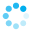# Calculating single transferable vote - positions that elect multiple candidates

This article will cover the process for how single transferable vote (STV) should be calculated.

###### When

All vote counts should be done by STV (single transferable vote). Online election uses this system.

For positions that elect multiple candidates, this generally means the quota needed to be elected of (number of votes cast in round) / (number of candidates including RON + 1).

Please note SVT is manageable to calculate by hand, but only for small elections. At the same time if too few voters, then ties are more likely. This is why we strongly recommend CSPs take part in our online run elections, as these calculations are done automatically.

##### Process

When no candidate reaches quota (a clear winner), the usual elimination process is done with votes redistributed on next preference and the vote goes to a second round.

When a candidate meets or exceeds quota in a round, that candidate is elected.

A quota redistribution takes place, where a fraction of the winning candidate's votes in excess of the quota are redistributed to other candidates, and the election continues to the next round to elect folowing candidates until all vacancies are filled.

###### An example of quota redistribution:

Say we have three candidates + RON, and we need to elect 2.

In round 1, 15 votes are cast, so the quota is 15 / (2 + 1) = 5. Let's image round 1 looks like this:

Candidate A: 10

Candidate B: 2

Candidate C: 2

RON: 1

Clearly Candidate A should be elected as they have exceeded quota by a wide margin. If we just ignore Candidate A for round 2 though, then the choice of who to elect out of candidates B & C is determined purely by the second preference of the single RON vote, and the second preferences of the 10 people who voted for Candidate A are ignored.

STV addresses this with a quota redistribution as follows:

Candidate A has 10 votes but only needs 5 to meet quota, so 10 - 5 = 5 votes can be redistributed. This is achieved by examining the second preferences of all 10 votes and redistributing a fraction of each vote by those second preferences. In this case, the fraction is 5 / 10 = 0.5, so that once redistributed Candidate A will retain 5 votes, and 5 votes will be redistributed to the remaining candidates. Let's imagine the second preferences of the 10 votes look like this:

Candidate B: 3

Candidate C: 4

RON: 2

No second preference: 1

The quota distribution will then look like this:

Candidate B: 2 + (3 x 0.5 )= 3.5

Candidate C: 2 + (4 x 0.5) = 4

RON: 1 + (2 x 0.5) = 2

Lost Votes: 0 + (1 x 0.5) = 0.5

In the second round, RON would then be eliminated, and votes redistributed (with care taken to redistribute the whole vote for the first preference received in R1 and the half votes for the second prefences received in R2). If we imagine for simplicity that none of the RON votes have any further preferences then neither of the remaining candidates receives any further votes and Candidate C is elected by simple majority in Round 3 (quota being 7.5 / 2 = 3.75, with Candidate C receiving 4 votes).

Elections FAQ

## Do you need help?

Loading, please wait